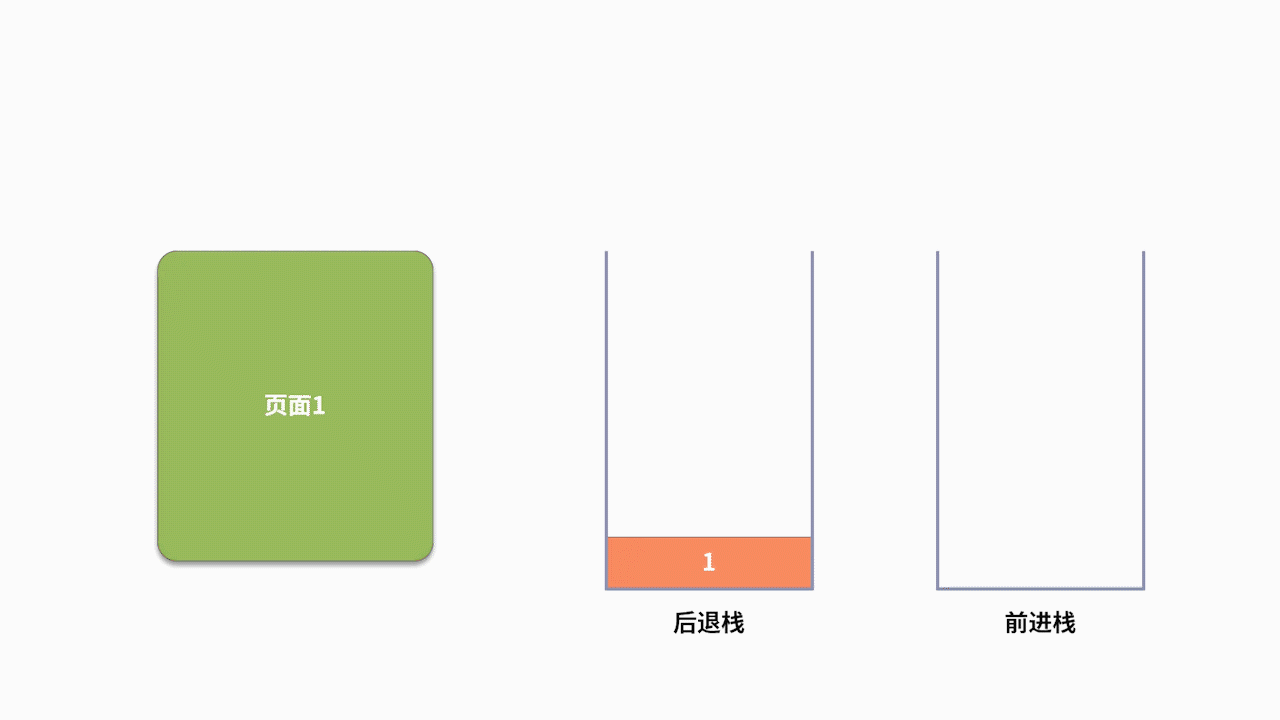05 栈：后进先出的线性表，如何实现增删查？

栈是什么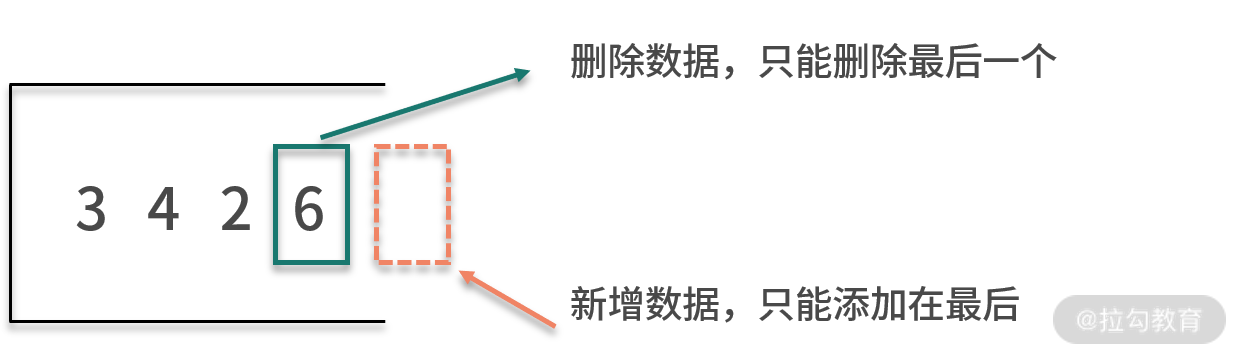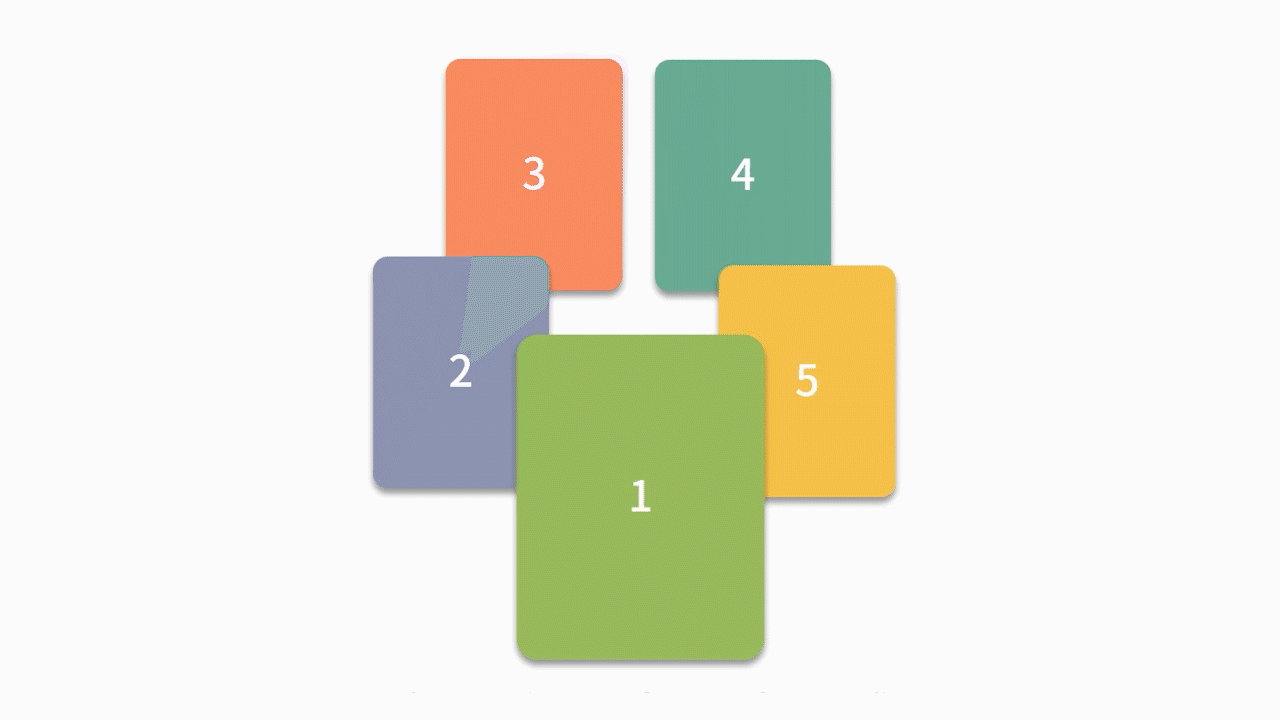栈的基本操作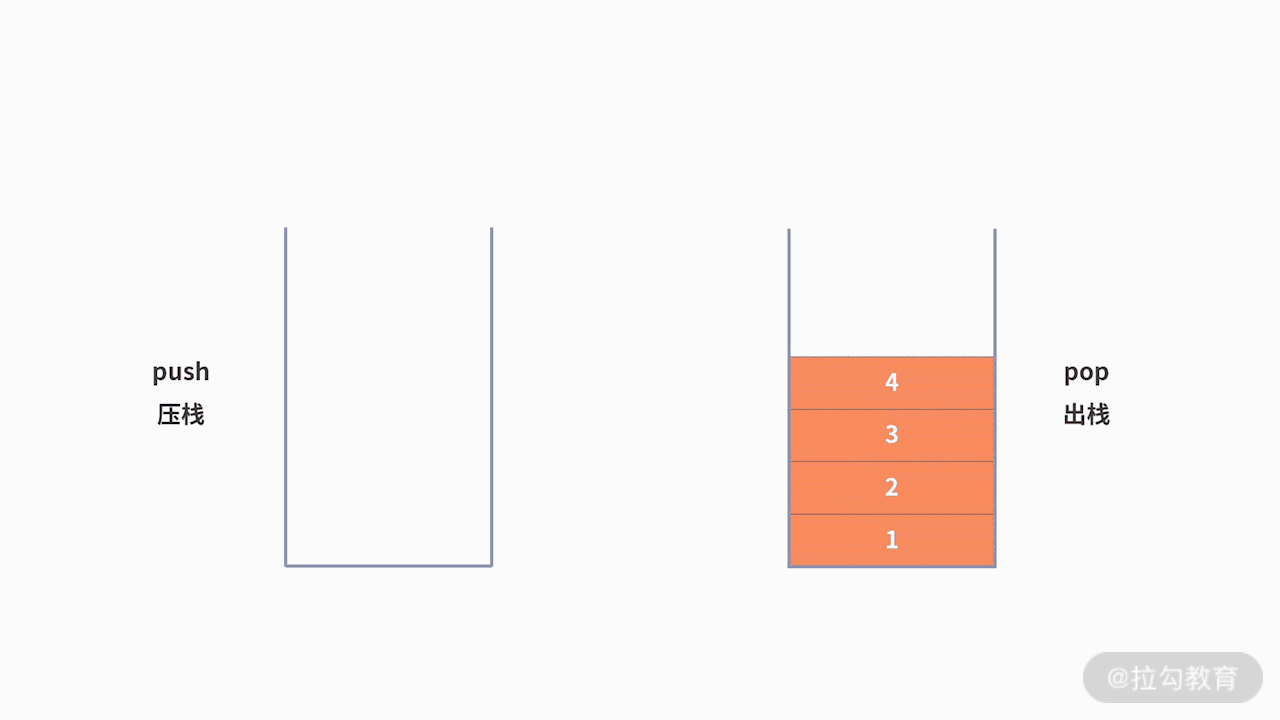顺序栈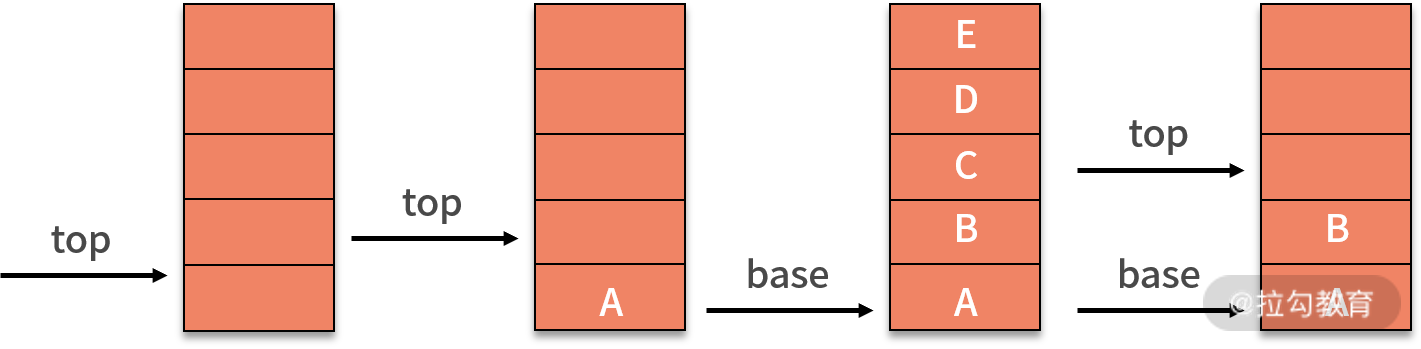链栈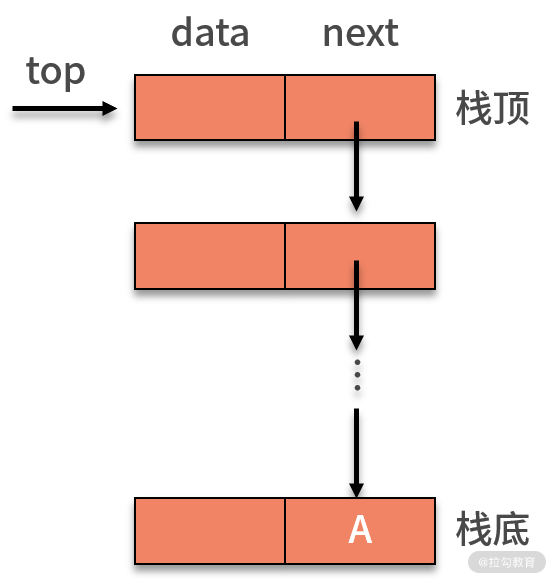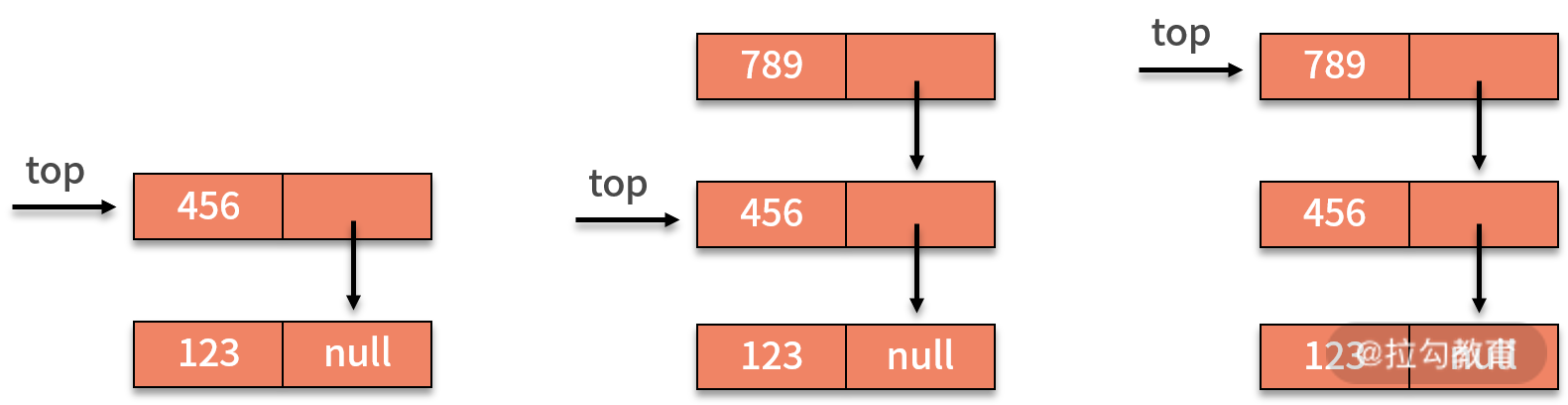栈的案例public static void main(String[] args) {
String s = "{[()()]}";
System.out.println(isLegal(s));
}
private static int isLeft(char c) {
if (c == '{' || c == '(' || c == '[') {
return 1;
} else {
return 2;
}
}
private static int isPair(char p, char curr) {
if ((p == '{' && curr == '}') || (p == '[' && curr == ']') || (p == '(' && curr == ')')) {
return 1;
} else {
return 0;
}
}
private static String isLegal(String s) {
Stack stack = new Stack();
for (int i = 0; i < s.length(); i++) {
char curr = s.charAt(i);
if (isLeft(curr) == 1) {
stack.push(curr);
} else {
if (stack.empty()) {
return "非法";
}
char p = (char) stack.pop();
if (isPair(p, curr) == 0) {
return "非法";
}
}
}
if (stack.empty()) {
return "合法";
} else {
return "非法";
}
}